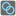• # ErrUchIsDown

## Question related to missionBreak Rings

I tried my code on my own computer runs well with just a bit long time (minutes if rings come to about 15), and when I run the code in checkio, "ErrUchIsDown" consistently appeared. Am I missing something? My codes:

import functools

import itertools

def break_rings(cons, cuts=1):

```    union = functools.reduce(lambda x,y:x|y, cons)
all_paths = list(itertools.permutations(union,cuts))
#print('all_paths:',len(all_paths))# a list of tuples
#print('Now {0} rings will be cut'.format(cuts))
input('continue')
result = []
path_no = 0
cons_remain = []
for path in all_paths:
temp_cons = list(cons)
count = 0
path_no += 1
#print('now taking path No.:', path_no)

for ring in path:
#print('now breaking ring:', ring)

if any((ring in i) for i in temp_cons):count += 1

#print('count', count)

for con in cons:
#print('Now temp_cons:',temp_cons)
if temp_cons == []:
return cuts
if len(con) > 1 and ring in con and con in temp_cons:
#print('Now try breaking con:',con)
#print(con,'is removed')
temp_cons.remove(con)

cons_remain.append(temp_cons)

if [] not in cons_remain: